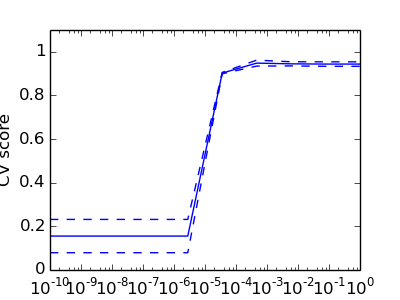This documentation is for scikit-learn version 0.16.1Other versions

If you use the software, please consider citing scikit-learn.

# Model selection: choosing estimators and their parameters¶

## Score, and cross-validated scores¶

As we have seen, every estimator exposes a score method that can judge the quality of the fit (or the prediction) on new data. Bigger is better.

```>>> from sklearn import datasets, svm
>>> X_digits = digits.data
>>> y_digits = digits.target
>>> svc = svm.SVC(C=1, kernel='linear')
>>> svc.fit(X_digits[:-100], y_digits[:-100]).score(X_digits[-100:], y_digits[-100:])
0.97999999999999998
```

To get a better measure of prediction accuracy (which we can use as a proxy for goodness of fit of the model), we can successively split the data in folds that we use for training and testing:

```>>> import numpy as np
>>> X_folds = np.array_split(X_digits, 3)
>>> y_folds = np.array_split(y_digits, 3)
>>> scores = list()
>>> for k in range(3):
...     # We use 'list' to copy, in order to 'pop' later on
...     X_train = list(X_folds)
...     X_test  = X_train.pop(k)
...     X_train = np.concatenate(X_train)
...     y_train = list(y_folds)
...     y_test  = y_train.pop(k)
...     y_train = np.concatenate(y_train)
...     scores.append(svc.fit(X_train, y_train).score(X_test, y_test))
>>> print(scores)
[0.93489148580968284, 0.95659432387312182, 0.93989983305509184]
```

This is called a KFold cross validation

## Cross-validation generators¶

The code above to split data in train and test sets is tedious to write. Scikit-learn exposes cross-validation generators to generate list of indices for this purpose:

```>>> from sklearn import cross_validation
>>> k_fold = cross_validation.KFold(n=6, n_folds=3)
>>> for train_indices, test_indices in k_fold:
...      print('Train: %s | test: %s' % (train_indices, test_indices))
Train: [2 3 4 5] | test: [0 1]
Train: [0 1 4 5] | test: [2 3]
Train: [0 1 2 3] | test: [4 5]
```

The cross-validation can then be implemented easily:

```>>> kfold = cross_validation.KFold(len(X_digits), n_folds=3)
>>> [svc.fit(X_digits[train], y_digits[train]).score(X_digits[test], y_digits[test])
...          for train, test in kfold]
[0.93489148580968284, 0.95659432387312182, 0.93989983305509184]
```

To compute the score method of an estimator, the sklearn exposes a helper function:

```>>> cross_validation.cross_val_score(svc, X_digits, y_digits, cv=kfold, n_jobs=-1)
array([ 0.93489149,  0.95659432,  0.93989983])
```

n_jobs=-1 means that the computation will be dispatched on all the CPUs of the computer.

Cross-validation generators
 KFold (n, k) StratifiedKFold (y, k) LeaveOneOut (n) LeaveOneLabelOut (labels) Split it K folds, train on K-1 and then test on left-out It preserves the class ratios / label distribution within each fold. Leave one observation out Takes a label array to group observations

ExerciseOn the digits dataset, plot the cross-validation score of a SVC estimator with an linear kernel as a function of parameter C (use a logarithmic grid of points, from 1 to 10).

```import numpy as np
from sklearn import cross_validation, datasets, svm

X = digits.data
y = digits.target

svc = svm.SVC(kernel='linear')
C_s = np.logspace(-10, 0, 10)
```

## Grid-search and cross-validated estimators¶

### Cross-validated estimators¶

Cross-validation to set a parameter can be done more efficiently on an algorithm-by-algorithm basis. This is why for certain estimators the sklearn exposes Cross-validation: evaluating estimator performance estimators that set their parameter automatically by cross-validation:

```>>> from sklearn import linear_model, datasets
>>> lasso = linear_model.LassoCV()
>>> X_diabetes = diabetes.data
>>> y_diabetes = diabetes.target
>>> lasso.fit(X_diabetes, y_diabetes)
LassoCV(alphas=None, copy_X=True, cv=None, eps=0.001, fit_intercept=True,
max_iter=1000, n_alphas=100, n_jobs=1, normalize=False, positive=False,
precompute='auto', random_state=None, selection='cyclic', tol=0.0001,
verbose=False)
>>> # The estimator chose automatically its lambda:
>>> lasso.alpha_
0.01229...
```

These estimators are called similarly to their counterparts, with ‘CV’ appended to their name.

Exercise

On the diabetes dataset, find the optimal regularization parameter alpha.

Bonus: How much can you trust the selection of alpha?

```from sklearn import cross_validation, datasets, linear_model